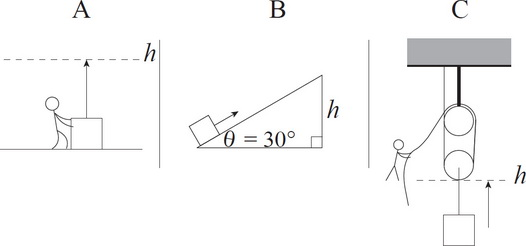# AP Physics 1 Question 320: Answer and Explanation

### Test Information

Question: 3208. The diagrams above show a box of mass m being lifted from the ground up to a height of h via three different methods. In situation A, the box is simply lifted by a person. In B, it is pushed up a ramp with an incline angle of 30 degrees. In C, it is lifted by a pulley system. Assuming ideal conditions (no friction) for all of these situations, which of the following correctly ranks the amount of work required to lift the box in each case?

• A. A > B > C
• B. A > B = C
• C. C > B > A
• D. A = B = C